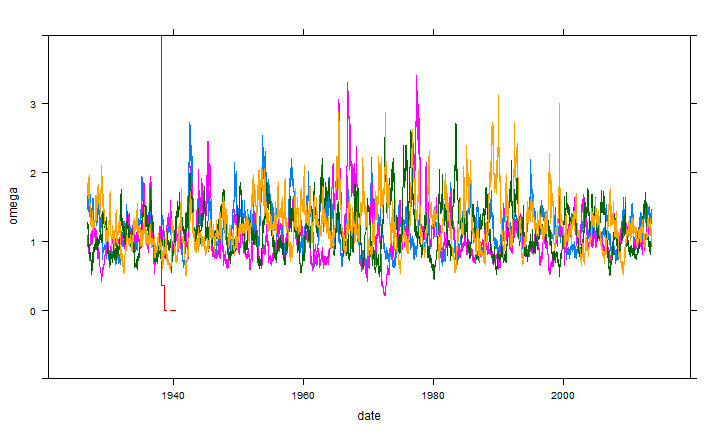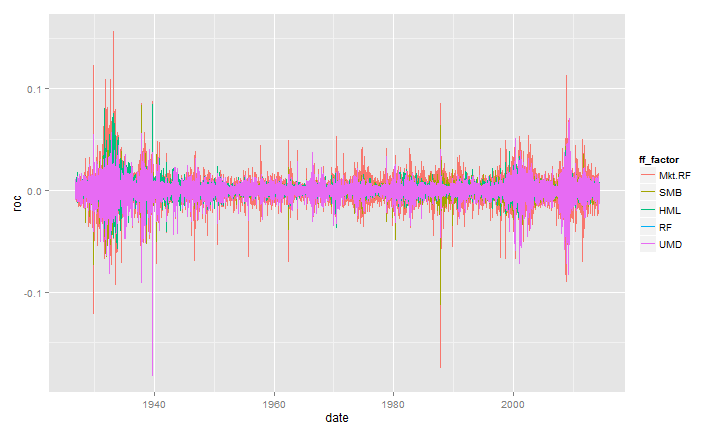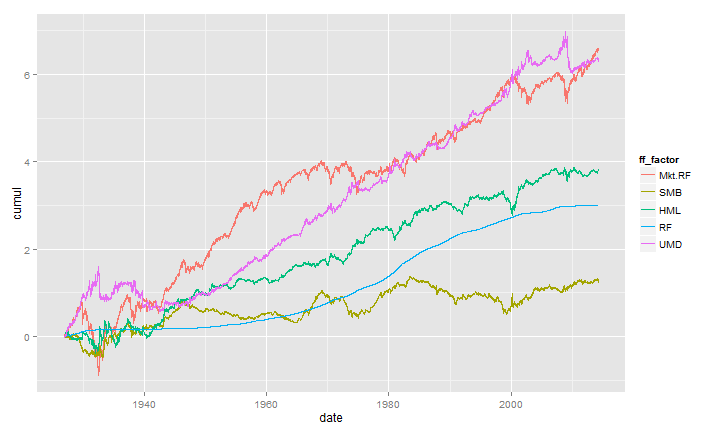# Rethinking R with Chains %>% tidyr + dplyr + magrittr + rCharts

Finance Case with French Factors

R seems to be experiencing a quiet revolution led by pipes | chains borrowed from Javascript, F#, and Unix. `dplyr` and `magrittr` are independent projects, but they have benefitted greatly from each other. Chaining results in much more readable code, and as a nice side benefit, Romain Francois' C magic makes `dplyr` extremely fast. I thought I would collect a couple of example workflows with the French-Fama factors and `xts` data. `dplyr` and `magrittr` are not designed to work with `xts` time series out of the box, so these time series require a couple of extra steps.

I will also use `tidyr`, which is Hadley Wickham's rethought `reshape2`. `tidyr` is designed to fit nicely into the `dplyr`/`magrittr` workflow. Its simplicity makes it power deceptive.

Best practices with chains in R are still not yet decided, and `magrittr` is evolving rapidly, so much might change, but I think we have already moved far enough in this direction that return to our old ways is unlikely.

Let's `require` all the libraries. If you do not have them, `install_github` from `devtools` will get you up to date.

``````require(quantmod)
require(PerformanceAnalytics)
require(dplyr)
require(tidyr)
require(magrittr)

#not necessary but include for examples
require(lattice)
require(ggplot2)
``````

Similar to lots of posts, I will use this ugly R code to load in the data from the Kenneth French data library.

``````#daily factors from Kenneth French Data Library
#get Mkt.RF, SMB, HML, and RF
#UMD is in a different file
my.url="http://mba.tuck.dartmouth.edu/pages/faculty/ken.french/ftp/F-F_Research_Data_Factors_daily.zip"
my.tempfile<-paste(tempdir(),"\\frenchfactors.zip",sep="")
my.usefile<-paste(tempdir(),"\\F-F_Research_Data_Factors_daily.txt",sep="")
quiet = FALSE, mode = "wb",cacheOK = TRUE)
unzip(my.tempfile,exdir=tempdir(),junkpath=TRUE)
#read space delimited text file extracted from zip
header = TRUE, sep = "",
as.is = TRUE,
skip = 4, nrows=23215)
#get xts for analysis
french_factors_xts <- as.xts(
french_factors,
order.by=as.Date(
rownames(french_factors),
format="%Y%m%d"
)
)

#now get the momentum factor
my.url="http://mba.tuck.dartmouth.edu/pages/faculty/ken.french/ftp/F-F_Momentum_Factor_daily.zip"
my.usefile<-paste(tempdir(),"\\F-F_Momentum_Factor_daily.txt",sep="")
quiet = FALSE, mode = "wb",cacheOK = TRUE)
unzip(my.tempfile,exdir=tempdir(),junkpath=TRUE)
#read space delimited text file extracted from zip
header = TRUE, sep = "",
as.is = TRUE,
skip = 13, nrows=23114)
#get xts for analysis
french_momentum_xts <- as.xts(
french_momentum,
order.by=as.Date(
rownames(french_momentum),
format="%Y%m%d"
)
)

#merge UMD (momentum) with other french factors
french_factors_xts <- na.omit( merge( french_factors_xts, french_momentum_xts ) )
french_factors_xts <- french_factors_xts/100
``````

I have noticed that rolling analysis with `xts` can sometimes be slow. `as.matrix` is my favorite way to speed things up, since I usually do not need `xts` powerful indexing and subsetting features. I thought the additional complexity of rolling analysis would offer a nice challenge to improve my understanding of `xts` + `dplyr`. Here is a quick test. I would love thoughts on a better approach with comments that offer the comprable `melt` and `ddply` method.

``````#now we should have all the french factor data that we need
#we can start to do our exploration
#but this time use dplyr
system.time(
df_dplyr <-
#get xts as data.frame to take advantage of new features
data.frame("date"=index(french_factors_xts),french_factors_xts) %>%
# long form similar to melt(
#  data.frame(
#    date=as.Date(index(french_factors_xts)),
#    french_factors_xts
#  ),
#  id.vars = "date",
#  variable.name = "mkt_factor",
#  value.name = "roc"
#)
gather(ff_factor,roc,-date) %.%
# group it and apply a function similar to ddply(
#   df,
#   .(ff_factor,roc),
#   summarise(
#     date = french_factors_xts\$date[seq(1,nrow(french_factors_xts)-199,by=1)],
#     omega = function(x) {
#       rollapply( as.numeric(x\$roc), Omega, width = 200, by = 1)
#     }
#   )
# )
group_by( ff_factor )  %.%
do(
data.frame(
date = .\$date[seq(1,nrow(.)-199,by=1)],
omega = rollapply( as.numeric(.\$roc) , Omega, width=200, by=1)
)
)
)
``````

|========= | 20% ~14 s remaining
|=================== | 40% ~10 s remaining
|============================= | 60% ~6 s remaining
|======================================= | 80% ~3 s remaining
Completed after 16 s
user system elapsed 15.74 0.02 16.28

This might be the longest I have gone without a plot, so let's use `lattice` to create a very quick and admittedly ugly line plot.

``````xyplot(omega~date, groups = ff_factor, data = df_dplyr,type="l",ylim=c(-1,4))
``````I am ashamed to admit how long it took me to realize that plotting could integrate nicely into chains. Below I show how we can use `Gmisc` `htmlTable` to nicely output a table with the last 5 daily returns from each of the factors.

``````require(Gmisc)
data.frame(
"date"=format(index(french_factors_xts)),
french_factors_xts
) %>%
gather(ff_factor,roc,-date) %>%
mutate(
date = as.character(date),
ff_factor = as.character(ff_factor),
roc = paste0(format(roc*100,digits=4),"%")
) %>%
group_by( ff_factor ) %>%
top_n(n=5,date) %>%
htmlTable %>%
cat
``````
. date ff_factor roc
1 2014-04-24 Mkt.RF 0.080%
2 2014-04-25 Mkt.RF -1.040%
3 2014-04-28 Mkt.RF 0.110%
4 2014-04-29 Mkt.RF 0.560%
5 2014-04-30 Mkt.RF 0.350%
6 2014-04-24 SMB -0.390%
7 2014-04-25 SMB -0.870%
8 2014-04-28 SMB -0.610%
9 2014-04-29 SMB -0.220%
10 2014-04-30 SMB 0.230%
11 2014-04-24 HML -0.080%
12 2014-04-25 HML 0.630%
13 2014-04-28 HML -0.430%
14 2014-04-29 HML -0.230%
15 2014-04-30 HML -0.030%
16 2014-04-24 RF 0.000%
17 2014-04-25 RF 0.000%
18 2014-04-28 RF 0.000%
19 2014-04-29 RF 0.000%
20 2014-04-30 RF 0.000%
21 2014-04-24 UMD -0.540%
22 2014-04-25 UMD -1.240%
23 2014-04-28 UMD -1.150%
24 2014-04-29 UMD 0.670%
25 2014-04-30 UMD 0.530%

I do not think it was intentional, but `ggplot2` also fits nicely and cleanly into our chains. Often, I think data cleaning and aggregation should be separated from the output, but it is nice to be able to walk from raw data to final output in one uninterrupted block of code.

``````data.frame("date"=index(french_factors_xts),french_factors_xts) %>%
gather(ff_factor,roc,-date) %>%
ggplot(data = .,aes(x=date,y=roc,colour=ff_factor)) + geom_line()
``````The previous plot did not do any calculations, so let's add a simple `cumsum` to get a cumulative line chart of the returns for each factor. These calculations could be much more complex using this same technique.

``````data.frame("date"=index(french_factors_xts),french_factors_xts) %>%
gather(ff_factor,roc,-date) %>%
group_by( ff_factor ) %>%
mutate(cumul = cumsum(roc)) %>%
ggplot(data = .,aes(x=date,y=cumul,colour=ff_factor)) + geom_line()
``````As the R world moves to chains and pipes, the entire vis world is simultaneously moving to interactive charts. Within R visualization, we can see this parallel shift to interactivity with `rCharts`, `ggvis`, `googleVis`, and `animint`. Since `ggvis` and `dplyr` share the same source, I am sure we will see `ggvis` chains soon, so here I will show `rCharts` in our chain.

``````require(rCharts)

data.frame(
"date"= french_factors_xts %>% index %>% format,
french_factors_xts,
row.names= NULL
) %>%
tbl_df %>%
gather(ff_factor,roc,-date) %>%
group_by( ff_factor ) %>%
mutate(cumul = cumsum(roc)) %>%
#demo filter to get end of month instead of daily
filter(
date %in% format(
index(
french_factors_xts[french_factors_xts %>% endpoints(on="months")]
)
)
) %>%
dPlot(
cumul~date
,groups="ff_factor"
,data = .
,type="line"
,xAxis = list(
, inputFormat = '%Y-%m-%d'
, outputFormat = "%b %Y"
)
,yAxis = list( outputFormat = ".2f")
)
``````

Refined output currently requires some additional manipulation. In the chart above, I do not like the x axis, and want to include some code to just make tick marks for each decade. For this to occur, `rCharts` functions might need to be redesigned to return the chart instead of manipulate the object. I will appeal to expert R gurus for the best approach to this. Here is my ugly first hack.

``````#very hacky way of accomplishing
#need to iterate to something better
modifyChartList <- function( x, element, val ) {
rTemp <- x\$copy()
rTemp[[element]] <- modifyList(rTemp[[element]], val)
return(rTemp)
}

data.frame(
#maybe chaining here makes more confusing
"date"= french_factors_xts %>% index %>% format,
french_factors_xts,
row.names= NULL
) %>%
tbl_df %>%
gather(ff_factor,roc,-date) %>%
group_by( ff_factor ) %>%
mutate(cumul = cumsum(roc)) %>%
#demo filter to get end of quarter instead of daily
filter(
date %in% format(index(french_factors_xts[french_factors_xts %>% endpoints(on="quarters")]))
) %>%
dPlot(
cumul~date
,groups="ff_factor"
,data = .
,type="line"
,xAxis = list(
, inputFormat = '%Y-%m-%d'
, outputFormat = "%b %Y"
)
,yAxis = list( outputFormat = ".2f")
) %>%
modifyChartList(
element = "templates",
val = list(afterScript = '
<script>
{{chartId}}.axes
.timePeriod = d3.time.years
.timeInterval = 10
{{chartId}}.draw();
</script>
'
)
)
``````

Fortunately a thoughtful reader commented with the better way to add `afterScript` using the `%T>%` operator from `magrittr`. I have modified the code from above with what I think is a better workflow which removes the need for our helper `modifyChartList`.

``````data.frame(
#maybe chaining here makes more confusing
"date"= french_factors_xts %>% index %>% format,
french_factors_xts,
row.names= NULL
) %>%
tbl_df %>%
gather(ff_factor,roc,-date) %>%
group_by( ff_factor ) %>%
mutate(cumul = cumsum(roc)) %>%
#demo filter to get end of quarter instead of daily
filter(
date %in% format(index(french_factors_xts[french_factors_xts %>% endpoints(on="quarters")]))
) %>%
dPlot(
cumul~date
,groups="ff_factor"
,data = .
,type="line"
,xAxis = list(
, inputFormat = '%Y-%m-%d'
, outputFormat = "%b %Y"
)
,yAxis = list( outputFormat = ".2f")
) %T>%
.\$setTemplate(afterScript = '
<script>
{{chartId}}.axes
.timePeriod = d3.time.years
.timeInterval = 10
{{chartId}}.draw();
</script>
'
)
``````

After a little bit of experimentation, chains and pipes quickly become quite natural. I will eagerly read any new code and closely follow `magrittr` to become even more skilled at this, so June 23, 2014 might be the last bit of code that I share with no chains.

As I hope you can tell, this post was more a function of the efforts of others than of my own.

Thanks specifically: